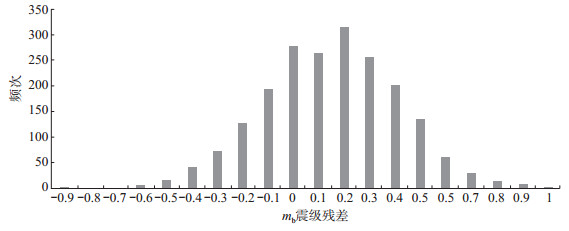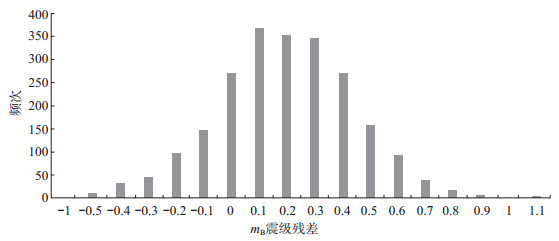﻿ 库尔勒地震台远震体波震级偏差分析文章快速检索
 地震地磁观测与研究2019, Vol. 40Issue (2): 34-38  DOI: 10.3969/j.issn.1003-3246.2019.02.0040

### 引用本文Li Xiaodong, Li Shanshan, Zhang Xiaofei, et al. Analysis of seismic magnitude deviation of Korla Seismic Station[J]. Seismological and Geomagnetic Observation and Research, 2019, 40(2): 34-38. DOI: 10.3969/j.issn.1003-3246.2019.02.004.### 文章历史

Analysis of seismic magnitude deviation of Korla Seismic Station
Li Xiaodong, Li Shanshan , Zhang Xiaofei , Zhou Yuanxin , Liu Qingshan , Duan Shaoxin
Korla Seismic Station, Xinjiang Uygur Autonomous Region 841000, China
Abstract: The digital seismic data were selected from 2008 to 2015 at Korla Seismic Station, comparing with the magnitude of body wave measured by China Earthquake Networks, the relationship between the magnitude, epicenter distance, focal depth and epicenter location was analyzed. According to the analysis results, the average deviation of mb is 0.145 5 and the average deviation of mB is 0.194 1, which indicates that the magnitude of the station calculation is relatively large. When the depth of the seismic source is 0-96 km, the magnitude deviation of mb and mB is close to the average. When the depth of the seismic source is h > 96 km, the measured magnitude is basically the same as that measured by China Earthquake Networks. When the earthquake occurs in the third quadrant, the measured magnitude of the station is basically the same as that measured by China Earthquake Networks.
Key words: body wave magnitude    station correction value    magnitude deviation    comparative analysis
0 引言

1 体波震级测定

 $m_{\mathrm{b}}或m_{\mathrm{B}}=\lg \left(\frac{A}{T}\right)_{\max }+Q({\mathit{Δ}}, h)+C$ (1)

2 体波震级偏差计算

 $E_{i}=M_{i}-M_{0 i}$ (2)

 $\Delta E_{i}=\frac{1}{N} \sum_{i=1}^{N}\left(M_{i}-M_{0 i}\right)$ (3)

 $\delta_{i}=\left[\frac{\sum_{i=1}^{N}\left(M_{i}-M_{0 i}\right)^{2}}{N-1}\right]^{\frac{1}{2}}$ (4)
2.1 mb震级偏差

 ${m_{{\rm{b台网}}}} = 0.7249{m_{{\rm{b台}}}} + 1.3530$图 1 库尔勒台记录mb震级偏差频次分布 Fig.1 Frequency distribution of mb magnitude deviation recorded by Korla Seismic Station表 1 mb台mb台网体波震级偏差统计 Tab.1 Statistical of magnitude deviation of body wave in mb台 and mb台网

2.2 mB震级偏差

 ${m_{{\rm{B台网}}}} = 1.0025{m_{{\rm{B台}}}} + 0.1802$图 2 库尔勒台记录mB震级偏差频次分布 Fig.2 Frequency distribution of mB magnitude deviation recorded by Korla Seismic Station表 2 mB台mB台网体波震级偏差统计 Tab.2 Statistical of magnitude deviation of body wave in mB台 and mB网台

3 震级偏差分析 3.1 震级大小与震级偏差之间的关系表 3 震级偏差值与震级大小关系 Tab.3 Relationship between magnitude and magnitude size deviation values
3.2 震中距与震级偏差的关系表 4 震级偏差与震中距的关系 Tab.4 The relationship between magnitude and epicentral distance
3.3 震源深度与震级偏差的关系表 5 震源深度与震级偏差的关系 Tab.5 The relationship between focal depth and the magnitude deviation
3.4 方位角与震级偏差的关系表 6 震级偏差与方位角的关系 Tab.6 The relationship between magnitude deviation and azimuth

4 结论

 刘红桂. 不同构造区固有吸收衰减和散射衰减相对强弱的对比研究[J]. 国际地震动态, 2012(1): 31-32. 刘瑞丰, 陈运泰, BormannP, 等. 中国地震台网与美国地震台网测定震级的对比(Ⅰ)——体波震级[J]. 地震学报, 2005, 27(6): 583-587. DOI:10.3321/j.issn:0253-3782.2005.06.001 任克新, 徐志国, 刘瑞丰, 等. IASPEI新标准体波震级分析研究[J]. 中国地震, 2009, 25(4): 377-385. DOI:10.3969/j.issn.1001-4683.2009.04.004 殷翔, 徐志国, 任枭, 等. 南京基准地震台与中国地震台网测定体波震级对比分析[J]. 地震地磁观测与研究, 2012, 33(2): 21-26. DOI:10.3969/j.issn.1003-3246.2012.02.004 中国地震局. 地震及前兆数字观测技术规范.地震观测(试行)[M]. 北京: 地震出版社, 2001: 12-13. 中国地震局监测预报司. 地震参数——数字地震学在地震预测中的应用[M]. 北京: 地震出版社, 2003: 10-11.